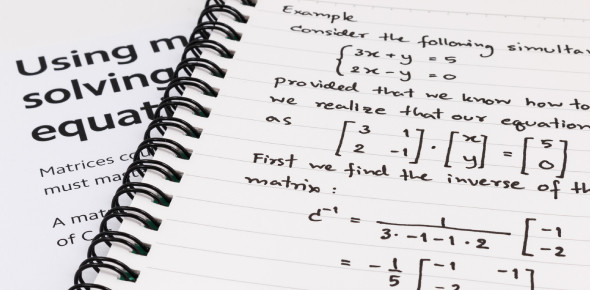# An Ultimate Matrices MCQ Quiz

30 Questions | Attempts: 1501
ShareSettingsDo you have any idea what is matrices in mathematics? Do you think you can pass this matrices quiz? A matrix is basically a rectangular collection or table of numbers, symbols, or expressions that are arranged in rows and columns in mathematics. Take this quiz and learn more about matrices in math. The order for seven matrices is described below. A is a 2x2 matrix. D is a 3x2 matrix. K is a 5x2 matrix. L is a 4x3 matrix. M is a 5x5 matrix. P is a 4x1 matrix. Q is a 1x5 matrix Use this information to answer the true/false statements.Back to top